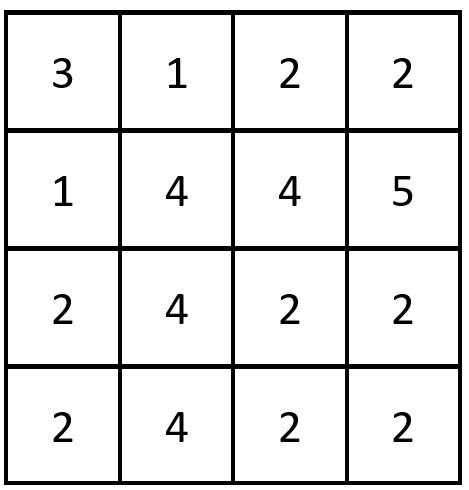2352. Equal Row and Column Pairs
Medium
497
21

Given a 0-indexed `n x n` integer matrix `grid`, return the number of pairs `(ri, cj)` such that row `ri` and column `cj` are equal.

A row and column pair is considered equal if they contain the same elements in the same order (i.e., an equal array).

Example 1:```Input: grid = [[3,2,1],[1,7,6],[2,7,7]]
Output: 1
Explanation: There is 1 equal row and column pair:
- (Row 2, Column 1): [2,7,7]
```

Example 2:```Input: grid = [[3,1,2,2],[1,4,4,5],[2,4,2,2],[2,4,2,2]]
Output: 3
Explanation: There are 3 equal row and column pairs:
- (Row 0, Column 0): [3,1,2,2]
- (Row 2, Column 2): [2,4,2,2]
- (Row 3, Column 2): [2,4,2,2]
```

Constraints:

• `n == grid.length == grid[i].length`
• `1 <= n <= 200`
• `1 <= grid[i][j] <= 105`
Accepted
38.1K
Submissions
54K
Acceptance Rate
70.6%

Seen this question in a real interview before?
1/4
Yes
No

Discussion (0)

Related Topics